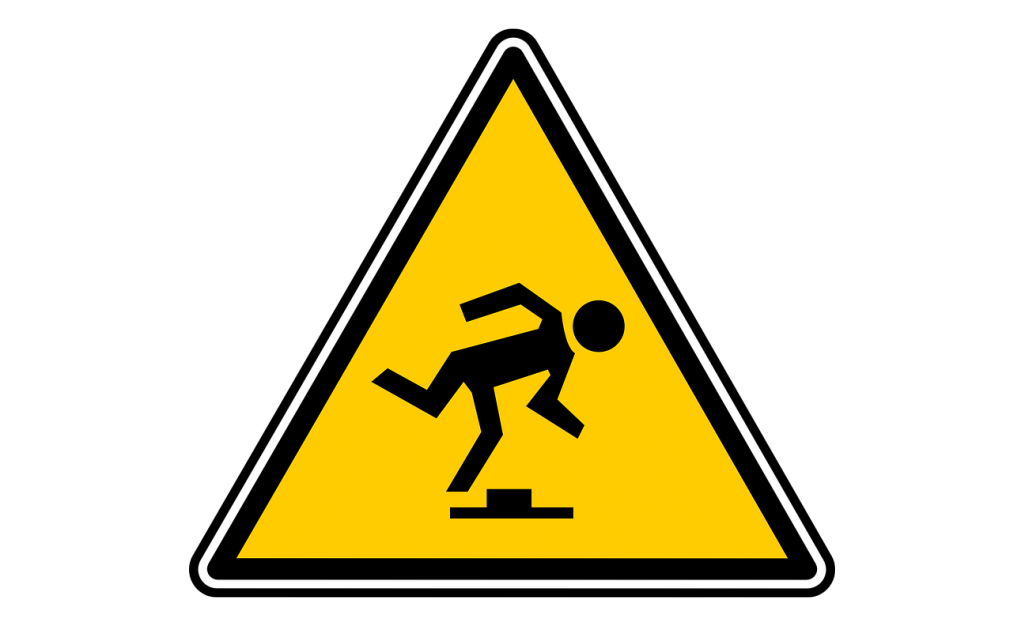# Grid Quantizer UnitIf you are looking for a pitch quantizer (i.e. a quantizer that knows about 1V/oct and scales) then see the Scale Quantizer Unit.

## Overview

This unit quantizes the incoming signal to a given number of discrete values (i.e. levels) with separate controls for pre-gain and post-gain.

The transfer function for when levels = 8.

The resulting mapping (or transfer function) is a staircase with stair height and frequency controlled by pre/post gain and level, respectively. Alternatively, when expressed in mathematical notation:

$\mbox{QUANTIZE}(x;\mbox{pre},\mbox{levels},\mbox{post}) = \frac{\mbox{FLOOR}(\mbox{levels} * \mbox{pre} * x) * \mbox{post}}{\mbox{levels}}$

Some applications are:

• adding grit to sounds (i.e. similar to bit crushing)
• restricting modulation signals to a finite set of levels (i.e. stair-case function)

## Parameters

### pre

This pre-gain multiplies the signal ‘'’before’’’ quantization.

### levels

This parameter sets the total number of output levels that exist between -0.5 and 0.5 (or equivalently, between 0 and 1). In other words, assuming the pre- and post-gains are 1, the distance between one output step and the next output step is: $$\frac{1}{\mbox{levels}}$$.

### post

This post-gain multiplies the signal after quantization.